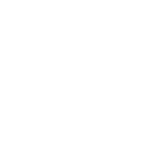Python

# Python Datetime Object Example

Hello in this tutorial, we will see how to use the Datetime module in python programming.

## 1. Introduction

A `datetime` package is an in-built python module that helps to encapsulate the date/time values. To dig digger in this tutorial we will need to download and install one extra dependency from the PyPi website. Use the `pip` command to install the `pytz` package.

Command

`pip install pytz`## 2. Python Datetime Object Example

Let us dive in with the programming stuff now. I am using JetBrains PyCharm as my preferred IDE. You’re free to choose the IDE of your choice.

### 2.1 Creating an implementation file

Let us understand the implementation with the help of a python script. The script explores different methods from the `datetime` module like fetching current date and timestamp, timezones, converting string timestamp to date-time object, etc.

Implementation file

```# python datetime object tutorial

# importing module
from datetime import datetime, timedelta, date

import pytz

# get current date and time
def get_current_date_time():
now = datetime.now()
print('Current date/time = {}'.format(now))

# get current date
def get_current_date():
now_date = date.today()
print('Current date = {}'.format(now_date))

# convert string to date time
def convert_string_to_datetime(date_str):
obj = datetime.strptime(date_str, '%B %d, %Y, %H:%M:%S')
print('Date = {}'.format(obj.date()))
print('Time = {}'.format(obj.time()))
print('Date = {}'.format(obj))

# get current date and time in utc
def get_current_date_time_in_utc():
now = datetime.now(pytz.utc)
print('Current date/time in utc = {}'.format(now))

# get timestamp from the given numeric timestamp
def get_timestamp_from_timestamp(timestamp):
obj = datetime.fromtimestamp(timestamp)
print('Date = {}'.format(obj))

# calculate the future date
def calculate_future_date(no_of_days):
now = datetime.now()
# calculating future date
future = now + timedelta(days=no_of_days)
print('Future date = {}'.format(future))

# driver code
if __name__ == '__main__':
# get_current_date_time()
print('----')

# get_current_date()
print('----')

# convert_string_to_datetime('November 5, 2020, 20:19:55')
print('----')

# get_current_date_time_in_utc()
print('----')

# get_timestamp_from_timestamp(1826254364)
print('----')

calculate_future_date(365)

print("\ndone")
```

You can uncomment the method of your choice and run this python script. If everything goes well the output will be shown in the IDE console.

Console output

```Current date/time = 2021-05-26 21:08:31.228270
----
Current date = 2021-05-26
----
Date = 2020-11-05
Time = 20:19:55
Date = 2020-11-05 20:19:55
----
Current date/time in utc = 2021-05-26 15:38:31.235326+00:00
----
Date = 2027-11-15 10:22:44
----
Future date = 2022-05-26 21:08:31.235326

done
```

That is all for this tutorial and I hope the article served you with whatever you were looking for. Happy Learning and do not forget to share!

## 3. Summary

In this tutorial, we learned:

• How to use the `datetime` package to manipulate date and time in python
• We explain through examples how this works

This was a tutorial to use the `datetime` package to manipulate date and time in python.

You can download the full source code of this example here: Python Datetime Object Example

Last updated on Jan. 21st, 2022

## Want to know how to develop your skillset to become a Java Rockstar?### Yatin

An experience full-stack engineer well versed with Core Java, Spring/Springboot, MVC, Security, AOP, Frontend (Angular & React), and cloud technologies (such as AWS, GCP, Jenkins, Docker, K8).
Subscribe
Notify ofThis site uses Akismet to reduce spam. Learn how your comment data is processed.